# testconsole.Results

(Removed) Gets results from test console simulations

## Compatibility

testconsole.Results has been removed. Use `comm.ErrorRate` or Bit Error Rate Analysis instead. For more information, see Compatibility Considerations.

## Description

The getResults method of the Error Rate Test Console returns an instance of a testconsole.Results object containing simulation results data. You use methods of the results object to retrieve and plot simulations results data.

## Properties

A testconsole.Results object has the properties shown in the following table. All properties are writable except for the ones explicitly noted otherwise.

PropertyDescription
TestConsoleNameError Rate Test Console. This property is not writable.
System Under Test NameName of the system under test for which the Error Rate Test Console obtained results. This property is not writable.
IterationModeIteration mode the Error Rate Test Console used for obtaining results. This property is not writable.
TestPointSpecify the name of the registered test point for which the results object parses results. The getData, plot, and semilogy methods of the Results object return data or create a plot for the test point that the TestPoint property specifies.
MetricSpecify the name of the test metric for which the results object parses results. The getData, plot, and semilogy methods of the Results object returns data or creates a plot for the metric that the Metric property specifies.
TestParameter1Specifies the name of the first independent variable for which the results object parses results.
TestParameter2Specifies the name of the second independent variable for which the results object parses results.

## Methods

A testconsole.Results object has the following methods.

### getData

`d = getData(r)` returns results data matrix, d, available in the results object r. The returned results correspond to the test point currently specified in the `TestPoint` property of r, and to the test metric currently specified in the `Metric` property of r.

If `IterationMode` is 'Combinatorial' then d is a matrix containing results for all the sweep values available in the test parameters specified in the `TestParameter1` and `TestParameter2` properties. The rows of the matrix correspond to results for all the sweep values available in `TestParameter1`. The columns of the matrix correspond to results for all sweep values available in `TestParameter2`. If more than two test parameters are registered to the Error Rate Test Console, d contains results corresponding to the first value in the sweep vector of all parameters that are not `TestParameter1` or `TestParameter2`.

If `IterationMode` is 'Indexed', then d is a vector of results corresponding to each indexed combination of all the test parameter values registered to the Error Rate Test Console.

### plot

`plot(r)` creates a plot for the results available in the results object r. The plot corresponds to the test point and test metric, specified by the `TestPoint` and `Metric` properties of r

If `IterationMode` is 'Combinatorial' then the plot contains a set of curves. The sweep values in TestParameter1 control the x-axis and the number of sweep values for TestParameter2 specifies how many curves the plot contains. If more than two test parameters are registered to the Error Rate Test Console, the curves correspond to results obtained with the first value in the sweep vector of all parameters that are not TestParameter1, or TestParameter2.

No plots are available when 'IterationMode' is 'Indexed'.

### semilogy

`semilogy(...)` is the same as `plot(...)`, except that the Y-Axis uses a logarithmic (base 10) scale.

### surf

surf(r) creates a 3-D, color, surface plot for the results available in the `results` object, r. The surface plot corresponds to following items:

• The test point you specify using the `TestPoint` property of the `results` object

• The test metric currently you specify in the `Metric` property of the `results` object

You can specify parameter/value pairs for the `results` object, which establishes additional properties of the surface plot.

When you select 'Combinatorial' for the IterationMode, the sweep values available in the test parameter you specify for the TestParameter1 property control the x-axis of the surface plot. The sweep values available in the test parameter you specify for the TestParameter2 property control the y-axis.

If more than two test parameters are registered to the test console, the surface plot corresponds to the results obtained with the parameter sweep values previously specified with the setParsingValues method of the results object.

You display the current parsing values by calling the getParsingValues method of the results object. The parsing values default to the first value in the sweep vector of each test parameter. By default, the surf method ignores the parsing values for any parameters currently set as TestParameter1 or TestParameter2.

No surface plots are available if the IterationMode is 'Indexed', when less than two registered test parameters exist, or TestParameter2 is set to 'None'.

### setParsingValues

```setParsingValues(R,'ParameterName1', 'Value1', ... 'ParameterName2', 'Value2', ...)``` sets the parsing values to the values you specify using the parameter-value pairs. Parameter name inputs must correspond to names of registered test parameters, and value inputs must correspond to a valid test parameter sweep value.

You use this method for specifying single sweep values for test parameters that differ from the values for TestParameter1 and TestParameter2. When you define this method, the `results` object returns the data values or plots corresponding to the sweep values you set for the setParsingValues method. The parsing values default to the first value in the sweep vector of each test parameter.

You display the current parsing values by calling the `getParsingValues` method of the results object. You may set parsing values for parameters in TestParameter1 and TestParameter2, but the results object ignores the values when getting data or returning plots.

Parsing values are irrelevant when `IterationMode` is 'Indexed'.

### getParsingValues

`getParsingValues` displays the current parsing values for the Error Rate Test Console.

`s = getParsingValues(r)` returns a structure, s, with field names equal to the registered test parameter names and with values corresponding to the current parsing values.

Parsing values are irrelevant when IterationMode is 'Indexed'.

## Examples

collapse all

Use the Bit Error Rate Analysis app to compute the BER as a function of ${\mathit{E}}_{\mathrm{b}}/{\mathit{N}}_{0}$. The app analyzes performance with either Monte Carlo simulations of MATLAB® functions and Simulink® models or theoretical closed-form expressions for selected types of communications systems. The code in the mpsksim.m function provides an M-PSK simulation that you can run from the Monte Carlo tab of the app.

Open the Bit Error Rate Analysis app from the Apps tab or by running the `bertool` function in the MATLAB command window.On the Monte Carlo tab, set the ${\mathbit{E}}_{\mathbf{b}}/{\mathbit{N}}_{0}$ range parameter to `1:1:5` and the Function name parameter to `mpsksim`.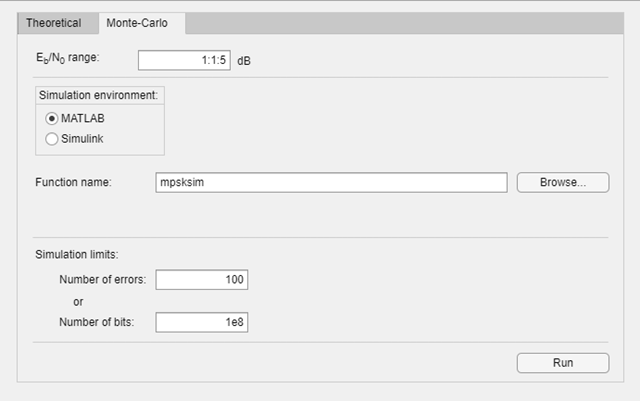Open the `mpsksim` function for editing, set `M=2`, and save the changed file.

Run the `mpsksim.m` function as configured by clicking Run on the Monte Carlo tab in the app.

After the app simulates the set of ${\mathit{E}}_{\mathrm{b}}/{\mathit{N}}_{0}$ points, update the name of the BER data set results by selecting `simulation0` in the BER Data Set field and typing `M=2` to rename the set of results. The legend on the BER figure updates the label to `M=2`.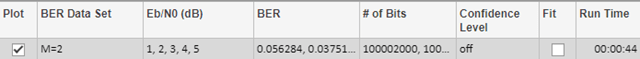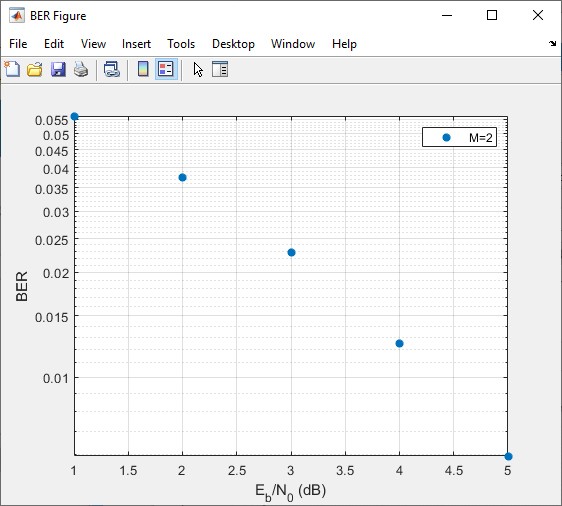Update the value for `M` in the `mpsksim` function, repeating this process for `M` = `4`, `8`, and `16`. For example, these figures of the Bit Error Rate Analysis app and BER Figure window show results for varying `M` values.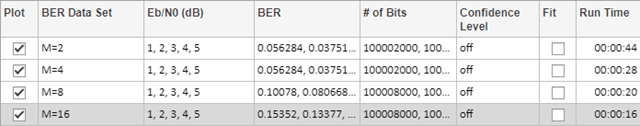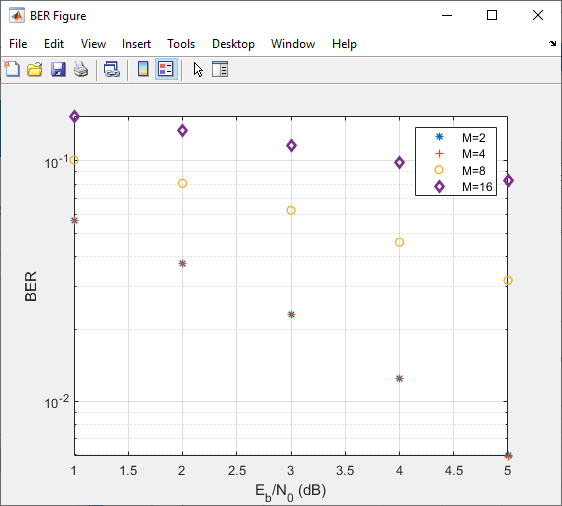Parallel SNR Sweep Using Bit Error Rate Analysis App

The default configuration for the Monte Carlo processing of the Bit Error Rate Analysis app automatically uses parallel pool processing to process individual ${\mathit{E}}_{\mathrm{b}}/{\mathit{N}}_{0}$ points when you have the Parallel Computing Toolbox™ software but for the processing of your simulation code: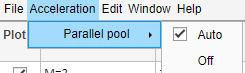The `commtest.ErrorRate` and `testconsole.Results` object packages will be removed in a future release. They can be used to perform parameter sweeps to analyze communication system performance. This example demonstrates a workflow that uses them and along with recommended alternate workflows.

Obtain bit error rate and symbol error rate of an M-PSK system for different modulation orders and EbNo values. System under test is `commtest.MPSKSystem`.

```% Create an M-ary PSK system systemUnderTest = commtest.MPSKSystem; % Instantiate an Error Rate Test Console and attach the system errorRateTester = commtest.ErrorRate(systemUnderTest); errorRateTester.SimulationLimitOption = ... 'Number of errors or transmissions'; errorRateTester.MaxNumTransmissions = 1e5; % Set sweep values for simulation test parameters setTestParameterSweepValues(errorRateTester,'M',2.^[1 2 3 4], ... 'EbNo',(-5:10)) % Register a test point registerTestPoint(errorRateTester,'MPSK_BER', ... 'TxInputBits','RxOutputBits') % Get information about the simulation settings info(errorRateTester)```
```Warning: commtest.ErrorRate will be removed in the future. Use comm.ErrorRate or bertool instead. See R2019b Communications Toolbox Release Notes for more information. Test console name: commtest.ErrorRate System under test name: commtest.MPSKSystem Available test inputs: NumTransmissions, RandomIntegerSource Registered test inputs: NumTransmissions Registered test parameters: EbNo, M Registered test probes: RxOutputBits, RxOutputSymbols, TxInputBits, TxInputSymbols Registered test points: MPSK_BER Metric calculator functions: @commtest.ErrorRate.defaultErrorCalculator Test metrics: ErrorCount, TransmissionCount, ErrorRate ```
```% Run the M-PSK simulations run(errorRateTester)```
```Starting parallel pool (parpool) using the 'local' profile ... Connected to the parallel pool (number of workers: 12). 12 workers available for parallel computing. Simulations will be distributed among these workers. Running simulations... ```
```% Get the results mpskResults = getResults(errorRateTester);```
```Warning: testconsole.Results will be removed in the future. See R2019b Communications Toolbox Release Notes for more information. ```
```% Get a semi-log scale plot of EbNo versus bit error rate for % different values of modulation order M mpskResults.TestParameter2 = 'M'; semilogy(mpskResults,'*-')```

Run an error rate simulation over M=2.^(1:4) and EbNo=-5:10. Use `comm.ErrorRate` to collect both bit error rate (BER) and symbol error rate (SER) data. Run the simulations to collect a minimum of 100 symbol errors or for a maximum of 1e5 symbols.

```% Set the M sweep values same as the commtest.ErrorRate object getTestParameterSweepValues(errorRateTester,'M')```
```ans = 1×4 2 4 8 16 ```
```MSweep = 2.^[1 2 3 4]; % Set EbNo sweep values same as the commtest.ErrorRate object getTestParameterSweepValues(errorRateTester,'EbNo')```
```ans = 1×16 -5 -4 -3 -2 -1 0 1 2 3 4 5 6 7 8 9 10 ```
```EbNoSweep = -5:10; % Set minumum number of errors same as the commtest.ErrorRate object errorRateTester.MinNumErrors```
```ans = 100 ```
```minNumErrors = 100; % Set maximum number of transmissions same as the commtest.ErrorRate % object. In this example a transmission is a symbol. errorRateTester.MaxNumTransmissions```
```ans = 100000 ```
```MaxNumTransmissions = 1e5; % Set frame length same as the commtest.ErrorRate object errorRateTester.FrameLength```
```ans = 500 ```
```frameLength = 500; % Find out if there is a parallel pool and how many workers are available [licensePCT,~] = license('checkout','distrib_computing_toolbox'); if (licensePCT && ~isempty(ver('parallel'))) p = gcp; if isempty(p) numWorkers = 1; else numWorkers = p.NumWorkers end else numWorkers = 1; end```
```numWorkers = 12 ```
```minNumErrorsPerWorker = minNumErrors/numWorkers; maxNumSymbolsPerWorker = MaxNumTransmissions/numWorkers; % Store results in an array, where first dimension is M and second % dimension is EbNo. Initialize the vector with NaN values. ser = nan(length(MSweep),length(EbNoSweep)); ber = nan(length(MSweep),length(EbNoSweep)); % First sweep is over M (modulation order) for MIdx = 1:length(MSweep) M = MSweep(MIdx); bitsPerSymbol = log2(M); % Second sweep is over EbNo for EbNoIdx = 1:length(EbNoSweep) EbNo = EbNoSweep(EbNoIdx); SNR = EbNo+10*log10(bitsPerSymbol); numSymbolErrors = zeros(numWorkers,1); numBitErrors = zeros(numWorkers,1); numSymbols = zeros(numWorkers,1); parfor worker = 1:numWorkers symErrRate = comm.ErrorRate; bitErrRate = comm.ErrorRate; while (numSymbolErrors(worker) < minNumErrorsPerWorker) ... || (numSymbols(worker) < maxNumSymbolsPerWorker) % Generate frameLength source outputs txMsg = randi([0 M-1],frameLength,1); % Modulate the data txOutput = pskmod(txMsg,M,0,'gray'); % Pass data through an AWGN channel with current SNR value chnlOutput = awgn(txOutput,SNR,'measured',[],'dB'); % Demodulate the data rxOutput = pskdemod(chnlOutput,M,0,'gray'); % Calculate number of symbol errors symErrVal = symErrRate(txMsg,rxOutput); numSymbolErrors(worker) = symErrVal(2); numSymbols(worker) = symErrVal(3); % Convert symbol streams to bit streams bTx = int2bit(txMsg,bitsPerSymbol); bRx = int2bit(rxOutput,bitsPerSymbol); % Calculate number of bit errors bitErrVal = bitErrRate(bTx,bRx); numBitErrors(worker) = bitErrVal(2); end end ber(MIdx,EbNoIdx) = sum(numBitErrors)/(sum(numSymbols)*bitsPerSymbol); ser(MIdx,EbNoIdx) = sum(numSymbolErrors)/sum(numSymbols); end end % Plot results semilogy(EbNoSweep,ber,'*-') grid on title('MPSK BER') xlabel('Eb/No') ylabel('BER') legendText = cell(length(MSweep),1); for p=1:length(MSweep) legendText{p} = sprintf('M: %d',MSweep(p)); end legend(legendText)```

## Version History

Introduced in R2009b

expand all

Errors starting in R2022a